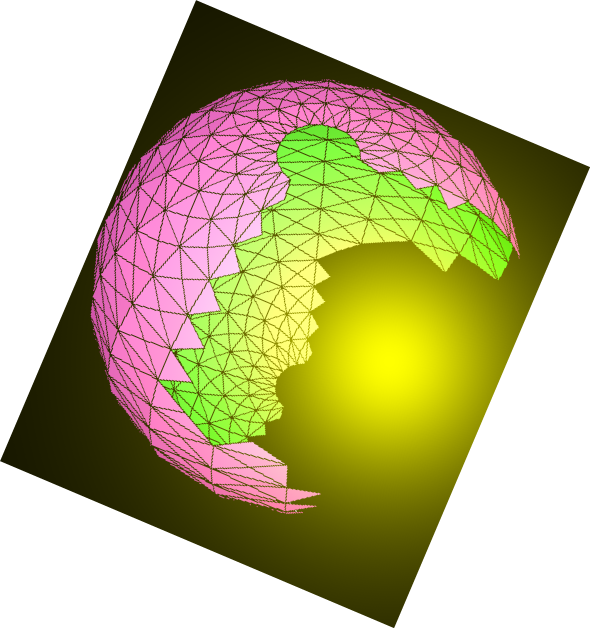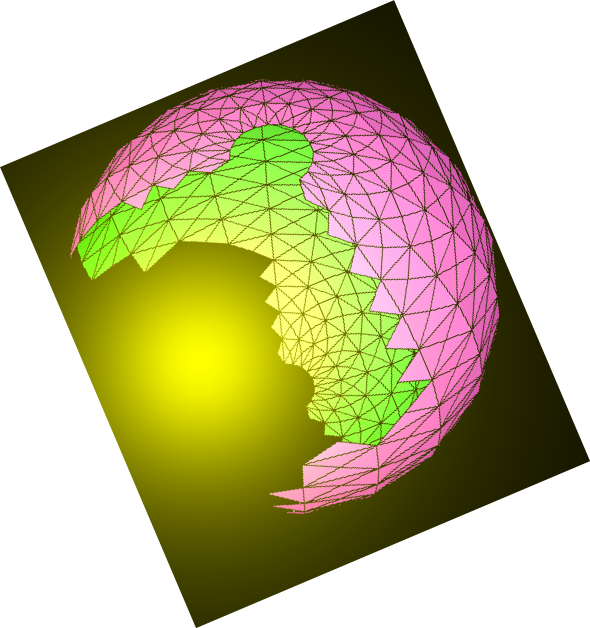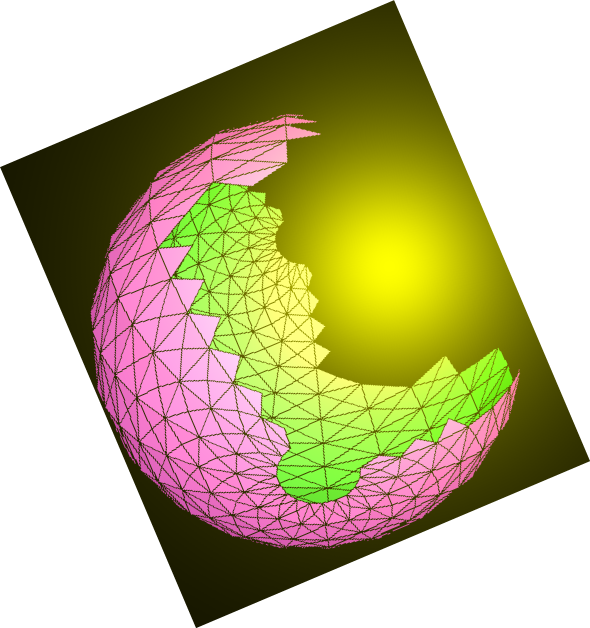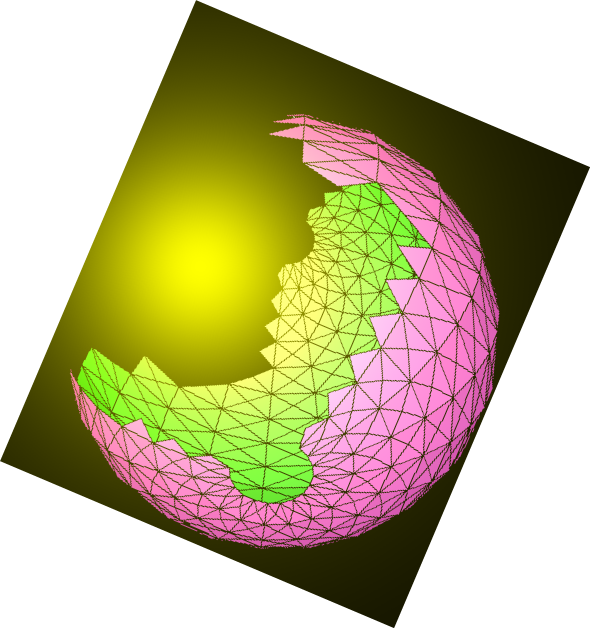# N.S.B. Cosmic Center# JavaScript & MathScript Interactive Document Generator-Demo-03

This document is generated by N.S.B. JavaScript & MathScript Interactive Document Generator version 001.

Introduction to N.S.B. JavaScript & MathScript Interactive Document Generator

JavaScript Data Types (cont.)

Like the number data type, the string data type is fundamental in JavaScript. Let us introduce a string and do some manipulations with it.

Consider the following:

###### output(1)=> Beauty is Eternity gazing at Herself in a Mirror!

The above string identified with the symbol s can be measured using the standard JavaScript length method:

###### output(2)=> 49

The above gives the number of characters in the string. In order to determine the character at a specific position in the string, the standard JavaScript notation is s[i], where i is an integer starting at 0 and ending at length-1. The following code defines an array, and fills it with the characters of the above string:

###### output(3)=> [ B, e, a, u, t, y, , i, s, , E, t, e, r, n, i, t, y, , g, a, z, i, n, g, , a, t, , H, e, r, s, e, l, f, , i, n, , a, , M, i, r, r, o, r, ! ]

In the following code, we construct a new string ss, copying the above characters, placing a plus sign between any two characters, and a double-plus between words.

###### output(4)=> B+e+a+u+t+y+++i+s+++E+t+e+r+n+i+t+y+++g+a+z+i+n+g+++a+t+++H+e+r+s+e+l+f+++i+n+++a+++M+i+r+r+o+r+!

Notice that the same JavaScript notation is used to get the characters of a string, like s[i], and the elements of an array, like aa[i].

In this program, we have many useful functions for identifying and dealing with characters in a string, as well as functions for dealing with substrings in a string. For example, the function isChrOfStr() checks whether a certain specific character is part of a specific string; the function countChrOfStr() tells you the number of times a specific character occurs in a specific string; the function indexChrOfStr() identifies the position of any such occurence.

Let's go back to our string s, and consider the following code sequence:

###### output(7)=> 5

Hence the character "i" is part of the string s, and occurs five times. We wish to know the position of the first occurence; but also other positions as well:

###### output(12)=> 0

You may notice from the above that the above functions consider the first character of a string to be at position 1. Notice as well, that if you ask for a position that does not occur, the answer is 0.

Likewise, we have similar functions to deal with substrings. Looking again at our string, consider the following code sequence:

###### output(17)=> 31

There are many other functions dealing with characters and strings. We may have examples of them in other demonstrative documents. Here we shall consider two more functions that are useful in constructing new strings from an existing one. These are takeStr() and dropStr(). With takeStr(), we may take a number of characters either from the beginning or from the end; likewise, with dropStr(), we may drop a number of characters from the beginning or from the end.

Going back to our original string, consider the following code sequence:

###### output(21)=> gazing at Herself

As you may have noticed, negative integers count from the end.

In the documents that follow, we shall consider some aspects of arrays and objects--two important data types of JavaScript. Later on we shall delve into MathScript, the more important feature of our program.

###### NextHome
N.S.B. Cosmic Center